## 5 Great Physics Problems: Formula-27 Solution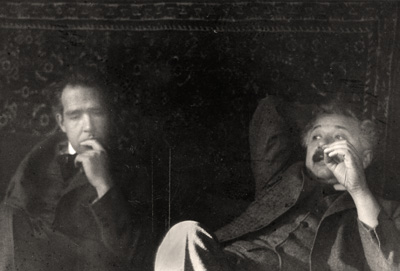Quantum mechanics and general relativity, still incompatible: No visible sight in finding a solution. This Mind-Being Paradigm is an attempt.

Five Great Problems in Theoretical Physics, According to Lee Smolin in his 2006 book The Trouble with Physics: The Rise of String Theory, the Fall of Science, and What Comes Next, Lee Smolin points out “five great problems in theoretical physics, expressed by Andrew Zimmerman Jones as follow. The Mind-Being Answers are expressed inside { }. For detail, see the previous seven Postings:

The problem of quantum gravity: Combine general relativity and quantum theory into a single theory that can claim to be the complete theory of nature. Quantum gravity is the effort in theoretical physics to create a theory that includes both general relativity and the standard model of particle physics. Currently, these two theories describe different scales of nature and attempts to explore the scale where they overlap yield results that don’t quite make sense, like the force of gravity (or curvature of spacetime) becoming infinite. (After all, physicists never see real infinities in nature, nor do they want to!)

{The big differences between Quantum Physics and General Relativity is that: Quantum non-locally past through two Mirrors to combine and form quantum objects; namely the Non-Local Psi and Psi* Mirrors. On the other hand General Relativity goes through only one Mirror, this is the non-local NOW mirror. All three of these Mirrors formed the Holon i—c—t or the 0—Infinity—0 Holon. This Holon non-locally connects the “Inside 0” —> White Hole, “Outside 0” —> Black Hole, and “Infinity = Circumference” —> Wormhole. Furthermore, this is a non-local teleporting Holon that connects Space–NOW–Infinity and thus resolved the Infinity in Relativistic Physics. In addition, Super-Symmetry applies only to Yin symmetry with Yang; hence Psi in symmetry with Psi*. This problem will be discuss below after answering all others first. The conservation through these three Mirrors is: d/dt (Information–Energy–Mass) = 0 rather than between Energy and Mass}

The Foundational Problems of Quantum Mechanics: One issue with understanding quantum physics is what the underlying physical mechanism involved is. The question that comes up in these interpretations revolves around what actually causes the collapse of the quantum wave function. (The puzzle of the curious aspect of human consciousness’s role in resolving these questions is related in Quantum Enigma.)

{The Observers Cause the Collapse; namely, Observer—Reference-Frame—Observed. What cause the collapse is the Being-In-Matter interacting with Holon of Spirit-Mind—Soul-Mind—Body-Mind of both Observer-Observed; This NOW interaction can be defined as a State of Consciousness. Its interaction is a non-local one and at the moment of NOW connecting Holon of 0—Infinity—0.}

The Unification of Particles and Forces: the standard model of particle physics shows a lot of different particles – 18 fundamental particles in all. Many physicists believe that a fundamental theory of nature should have some method of unifying these particles….

{their unification is with yin-yang line-diagram logic, while the leptons and force logic particles are unified (or encoded) with yin—yang—neutral line-diagrams. Here neutral line has to do with denoting free wills in picking yin or yang lines or diagrams. It is free will that cause these invisible unseen truth of Information particles to become visible energy-mass particles}

Einstein’s famous question “Did God have any choice when he created the universe?” Do the properties of the universe inherently set the form of the universe, because these properties just won’t work if the form is different? The answer to this seems to be leaning strongly toward the idea that there is not only one universe that could be created, but that there are a wide range of fundamental theories (or different variants of the same theory, based on different physical parameters, original energy states, and so on) and our universe is just one of these possible universes.

{But God had choosing these logic to create His Universe, the Logic set are #9 in Base-9, as such 1+2+3+4+5+6+7+8 = 9 +0 = 9 formed inside a set of Pre-Heaven Octagon Logic, and God also gave to all His Creation Free Will for their own creations. Thus, the God’s Universe is the beginning of the many Universes created by Ascended Humans with their free wills. These Universes can therefore be referred to as Co-Created Universes. Thus, the Co-Created Universes would have the same sets of Pre-Heaven / Post-Heaven Logic Inputted by God, but rearranged with the intentions of the Ascended.}

The tuning problem: In this case, the question becomes why our universe has properties that seem to be so finely tuned to allow for the existence of life. This question is called the fine-tuning problem and has promoted some physicists to turn to the anthropic principle for explanation, which dictates that our universe has the properties it does because if it had different properties, we wouldn’t be here to ask the question.

{There are no continuous tuning since God arrange all the universes’ input-output through precise executions inside the Vacuum Computer formed by the encoded line logic of the Pre-Post Heaven Octagons. The Ascended individual’s universe is also Co-Created inside the Vacuum Computer with one’s own arrangement of each sets of these two Octagons. The precision of these creations are due to the pre-heaven and post-heaven constants that Bind with constant 137, which also Bind Being to Matter through the states of Being-In-Matter.}

The Problem of Cosmological Mysteries: The universe still has a number of mysteries, but the ones that most vex physicist are dark matter and dark energy. This type of matter and energy is detected by its gravitational influences, but can’t be observed directly, so physicists are still trying to figure out what they are.

{Dark matter —> unseen Truth, #27, the Logic-Universe; while Dark energy —> Visible Truth, #37, forming the Energy-Universe. These two Universes are connected by a common Universe of Information. Dark matters in the Logic Universe are referred to as the Logic-Masses. The connecting Universe contains  Information-Masses, which are continuously in a state of Becoming. The Logic-Masses are encoded by the Yin-Yang Line-Diagrams, while the Information-Masses are encoded by the Yin-Yang-Neutral Line Diagrams. Outputs of the Information-Masses from the Vacuum Computer are the Energy-Masses of the Physical Universe. Logic-Masses are encoded logic inside the Computer. Information-Masses are executing mass-variables inside the computer. The Computer’s Outputs, namely the Energy-Masses become TRUTH, #64.}

THE THREE MIRRORS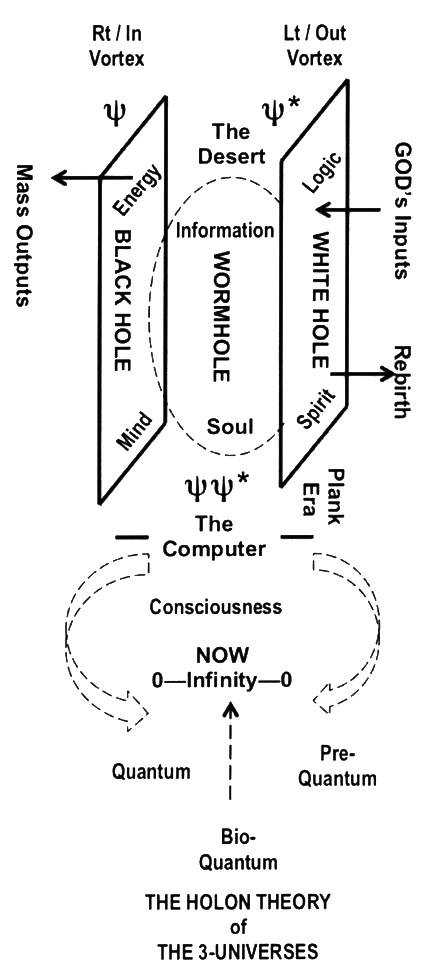AND THE DIAGRAM FOR THE LOGIC BIG BANG AND THE ENERGY BIG BANG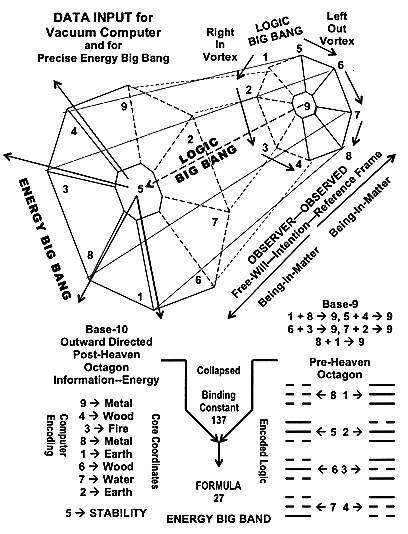The Right Side Base-9 Logic set is God’s Input. The Left-Side Base-10 Logic set is the arranged Computer encoded-decoded Logic.

Re-Illustrate the NDE diagram from previous postings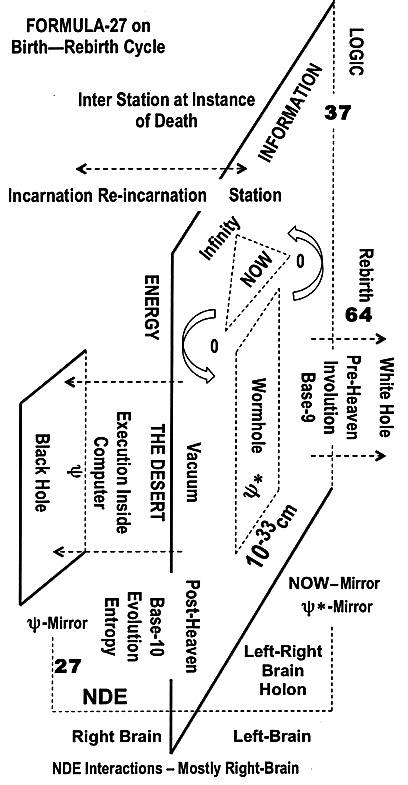The remaining part of Question 1. Using Super-Symmetry on Gravity, its force becomes infinite. like the force of gravity (or curvature of spacetime) becoming infinite. (After all, physicists never see real infinities in nature, nor do they want to!)

THE MIND-BEING PARADIGM’S VIEW OF SUPER-SYMMETRY

TWO MIRRORS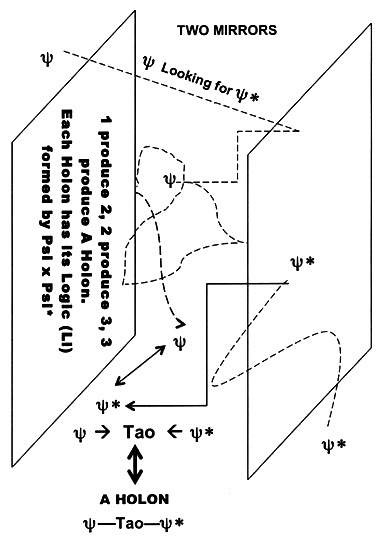EXAMPLE OF THIS HOLON IS SHOWN BELOW

COMPARES TO ONE MIRROR GRAVITY

GRAVITY MIRROR

0—Infinity—0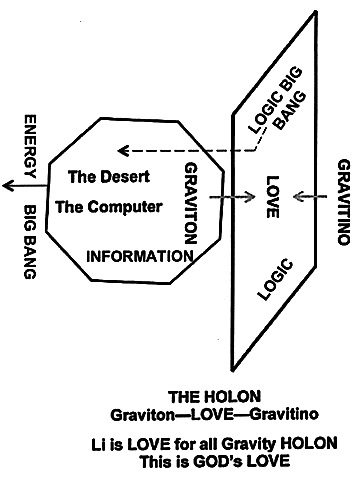Again the Gravity Mirror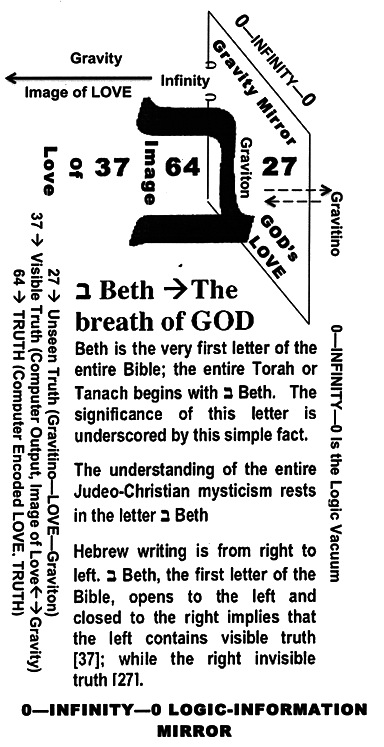There are a big differences between One Mirror and Two Mirror. In the case of Gravity, the Li is GOD given LOVE that explain the weakness of its “force-logic” (see the above Mirror Diagram). Remember

1 / Infinity –> Imaginary i, The Teleport Operator between Logic-Information and Information-Energy / Energy-Mass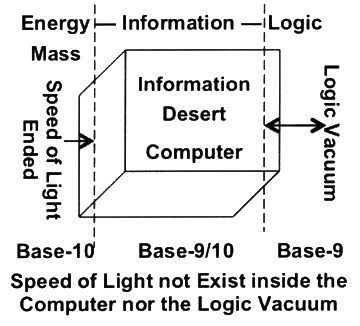On the other hand the two-Mirror case, the Li is not GOD given. Their success of finding the Li depends a lot of “hard works”, as shown in the above Two- Mirror diagram and the one below.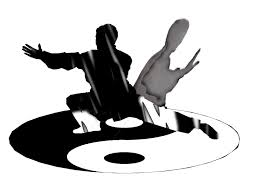As shown, the Li connects the one with his double as in the TAI-CHI / YIN-YANG Movement Diagram. The Li (or Logic) is the left-right figures formed a continuous PHI-SPIRAL inside two META-CUBES as shown in the left-diagram below. The middle of the Phi-Spiral is at the Navel (better shown on the right). Together, forms the Holon of three META-CUBES. The key here is that the upper half indicates Base-9 (Pre-Heaven) Logic and the bottom (Post-Heaven) Base-10 logic. Base-9 —-> Logic Big Bang, Base-10 —-> Energy Big Bang. The Third META-CUBE formed the Informational transitions. In the Tai-Chi Diagram, the movements simulate the Super-Symmetry between Heaven and Earth with Man at the middle. This is the Li in Tai-Chi Movements.

PARADIGM SUMMARY: WITH GOD FORMULATING THE INPUTS TO START THE UNIVERSE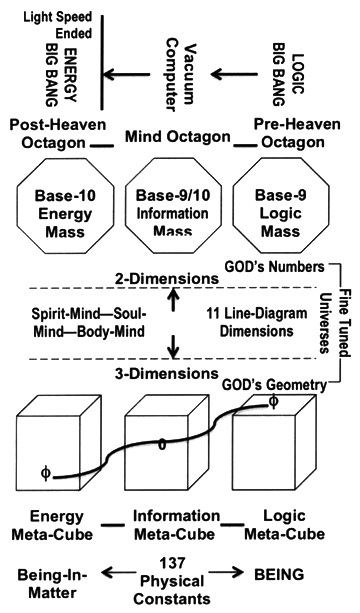AND THE THREE OCTAGON HOLON; PRE—POST—MIND AS SHOWN BELOW